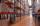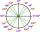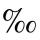Comparing powers

How many times is number 56 larger than number 46?

Result

x =  3.81

Solution:Leave us a comment of example and its solution (i.e. if it is still somewhat unclear...):Be the first to comment!Next similar examples:

1. Compare IIWhich of the numbers 710, 107 is greater?
2. LogisticsHead of logistics department informed the meeting of management of the same item is currently not available in sufficient quantity in all three warehouses of the company. We have the total 135 pieces. It was 37 pieces more in the warehouse No. 3 than in thWhat is greater angle? -0.7 radians or -0.7π radians?
4. PowersExpress the expression ? as the n-th power of the base 10.
5. EquationHow many real roots has equation ? ?
6. Volleyball8 girls wants to play volleyball against boys. On the field at one time can be six players per team. How many initial teams of this girls may trainer to choose?
7. PermillHow many permill is 978 from 84370?
8. Factory and divisionsThe factory consists of three auxiliary divisions total 2,406 employees. The second division has 76 employees less than 1st division and 3rd division has 212 employees more than the 2nd. How many employees has each division?
9. PowerNumber ?. Find the value of x.
10. One halfOne half of ? is: ?
11. Theorem proveWe want to prove the sentence: If the natural number n is divisible by six, then n is divisible by three. From what assumption we started?
12. Linear systemSolve a set of two equations of two unknowns: 1.5x+1.2y=0.6 0.8x-0.2y=2
13. Functions f,gFind g(1) if g(x) = 3x - x2 Find f(5) if f(x) = x + 1/2
14. 6 termsFind the first six terms of the sequence. a1 = 7, an = an-1 + 6
15. TreesA certain species of tree grows an average of 0.5 cm per week. Write an equation for the sequence that represents the weekly height of this tree in centimeters if the measurements begin when the tree is 200 centimeters tall.
16. Nineteenth memberFind the nineteenth member of the arithmetic sequence: a1=33 d=5 find a19
17. Two equationsSolve equations (use adding and subtracting of linear equations): -4x+11y=5 6x-11y=-5